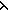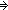IF YOU CAN SEE THIS go to /sfa/Nuprl/Shared/Xindentation_hack_doc.html

### Multi-argument Recursive Functions - continued from Y-combinator Continued

We have seen how one-argument recursive functions can be defined via the Y-combinator.

Consider now the form:

f(xyz) == G(xyzu,v.f(uyv))

Here we have introduced a couple of complexities. First, there are three arguments, but second, notice that the middle argument is held constant during recursion.

The system converts this recursive definition form to the following explicit form of definition using the Y-combinator:

f(abc) == Y((h,x,z. G(xbzu,v.(h(u,v)))),a,c)

Notice that the function given as argument to Y has a place h for the recursion, and places x and z holding places for new values of the first and third arguments on recursive calls. But there is no way for the second argument to be changed on recursion -- it is held at b, and so is not supplied as an argument to Y(h,x,z. G(xbzu,v.(h(u,v)))) on the right hand side like a and c are.
Here's how f(abc) evaluates after expanding the above definition (recall "g(r,s,t)" is really "g(r)(s)(t)"):

Y((h,x,z. G(xbzu,v.(h(u,v)))),a,c)
*(h,x,z. G(xbzu,v.(h(u,v))))(Y(h,x,z. G(xbzu,v.(h(u,v)))),a,c)
*G(abcu,v.(Y((h,x,z. G(xbzu,v.(h(u,v)))),u,v)))

The fixed-point Y(h,x,z. G(xbzu,v.(h(u,v)))) gets replicated during evaluation.

(Feb 2001 - sfa )